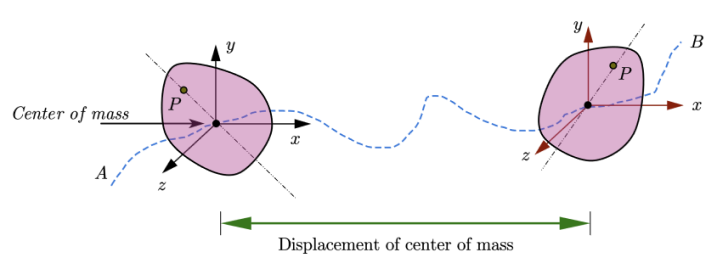# Rotational Motion

## Introduction to rotational motion

### What is rotational motion

Pure Rotational motion is the motion of a body about a fixed axis. If a rigid body is moved in such a way such that all the particles constituting it undergoes circular motion about a common axis then that type of motion is rotational motion.

Rotational motion of a rigid body around a fixed axis is a special case of rotational motion. During rotational motion there is also a possibility of axis changing its orientation. We can not explain the concepts of wobbling or precession by using fixed axis hypothesis for rotational motion.(wikipedia)

Here in this article about rotational motion I am assuming that you are comfortable in describing motion in physics. In addition to this you are also aware of types of motion in physics.. After completing this lesson you would be able to explain Rotational motion and know the difference between rotational motion and circular motion. We will also look at rotational motion examples from activities around us in our daily life.

Till now in our study of force and laws of motion we have always analyzed motion of an object by considering it as a particle even when the size of the object is not negligible. In this process we represent object under consideration as a point mass and shape and size of the object remains irrelevant while discussing the particular problem under consideration. Here in the study of rotational mechanics, this point mass or point particle model is inadequate for problems involving rigid body motion i.e. rigid body undergoing both translational motion and rotational motion. One more reason for not considering the body as particle is that all the particles of the body do not undergo same linear displacement.

As an example consider the motion of a wheel, we cannot consider a wheel as a single particle because different parts of the wheel in motion has different velocities and acceleration.
Here in rotational mechanics, we will consider rigid bodies and their motion. Rigid bodies have definite shape and size and they are capable of having both rotational and translational motion.

What is a rigid body?
The rigid body is a body with a perfectly defined and unchanging shape that is no matter how the body moves, the distance between any two particles within the body remains constant.
Although the way we define rigid body gives us the definition of an idealized rigid body and real materials always deforms on the application of force and this idealized rigid body assumption can be used freely for the substances where deformation is negligibly small and can be neglected.

Motion of a rigid body in general can be considered to consist of a translation of center of mass of the body plus rotation of the body about an axis through the center of mass as shown below in the figure:Figure: Motion of a rigid body having transnational motion and rotational motion about center of mass along path $AB$. You could notice the change in angular position of Point $P$ in between two positions of the moving body.

Get rotational motion formulas list by following this link.

## Rotational motion Examples

We see rotational motion examples in our daily life. Some of the examples of rotational motion are

1. Rotation of earth about its own axis create the cycle of day and night.
2. Motion of wheel, gears, motors, etc is rotational motion.
3. Motion of the blades of the helicopter is also rotatory motion.
4. A door, swiveling on its hinges as you open or close it.
5. A spinning top, motion of a Ferris Wheal in an amusement park.

If you want to learn more about rotational dynamics and clear your concepts further then there is a very good book by MTG Interactive Physics: Rotational Dynamics where you can clear your concepts further and gain knowledge accordingly.
For those of you who are interested in video tutorials can look at this video course by udemy
Mastering Rotational Kinematics and Dynamics
In this course, you can learn concepts like Angular variables, Concept of Angular Velocity and Acceleration, Rolling, Rotation and Torque, Mechanics of a Rolling Mass on an Inclined Plane, Physics behind a YOYO and other such related concepts. What I like about this course is how the instructor builds concepts of rotational mechanics as he takes the course further. There are enough solved examples for students which help them improve their problem-solving skills.